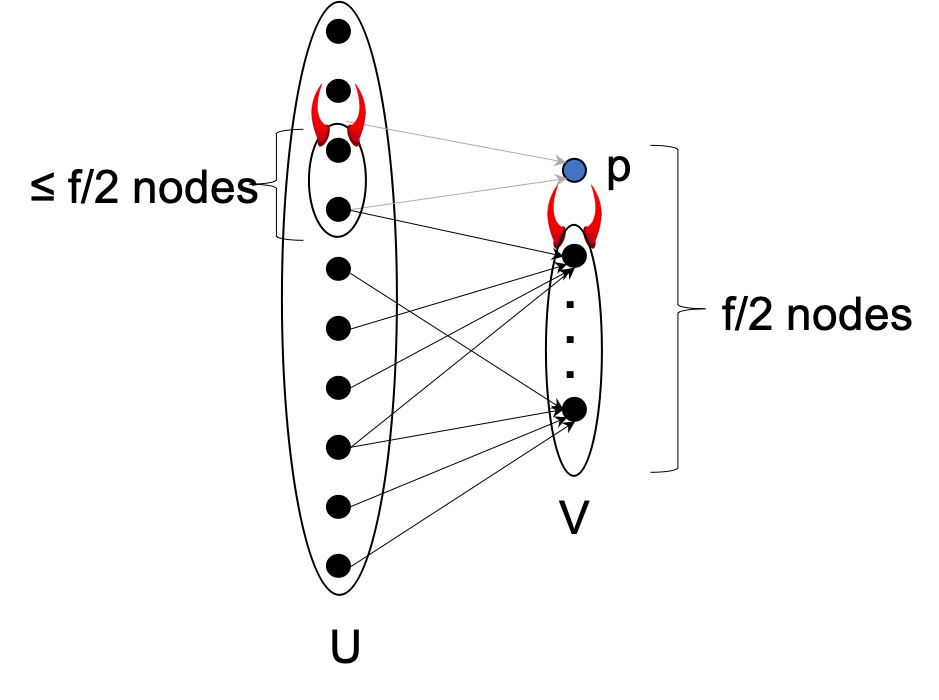The quest for building scalable Byzantine agreement has many challenges. In this post we highlight the 1982 Dolev and Reischuk lower bound.

In this series of posts we are revisiting classic lower bounds from the 1980’s. Most of them focused on deterministic protocols and computationally unbounded adversaries. Part of our goal is to provide a more modern view that also considers randomized protocols instead.

In our earlier lower bound posts, we discussed the limits on adversarial threshold (i) DLS, under partial synchrony, and (ii) FLM, in the absence of a trusted setup. In this post, we discuss yet another classic impossibility result on the limits on communication complexity in Byzantine Broadcast.

Dolev and Reischuk 1982: if there are $f$ corruptions, any deterministic Byzantine Broadcast protocol needs to send $> (f/2)^2$ messages.

In 1980, PSL showed the first feasibility result for consensus in the presence of Byzantine adversaries. However, their solution had an exponential (in $n$, the number of parties) communication complexity. An obvious question then is to figure out the lowest communication complexity that could be obtained. Dolev and Resichuk showed that the barrier to quadratic communication complexity cannot be broken by deterministic protocols.

At a very high level, the Dolev and Resichuk lower bound says that if you send few messages $< (f/2)^2$, then some honest party will receive no message! The party that receives no message has no way of reaching agreement with the rest. We use a trivial indistinguishability argument and create a world where a node $p$ receives no message at all. Thus, node $p$ cannot distinguish between a world where the designated sender sends 0 vs a world where it sends 1.

Here’s the proof intuition: In any set of $f/2$ nodes, if all of these nodes receive $> f/2$ messages from honest nodes, then we have a protocol with $> (f/2)^2$ messages. So, if there exists a protocol sending fewer messages, there must exist one node, say node $p$, that receives $\leq f/2$ messages. Now imagine that all of the nodes sending messages to node $p$ (there can be at most $f/2$ of them) are corrupt. If these corrupt nodes do not send anything to node $p$, then it may output a value that is not the same as what other honest nodes output, thereby causing a consistency violation.

Now let us formalize this intuition to ensure that the remaining honest nodes do not send a message to node $p$ to help $p$ output the correct value. This may be possible, for instance, if node $p$ contacts all other nodes. We will prove the theorem by describing two worlds and using indistinguishability for all honest nodes. Here we go.

World 1:In World 1, the adversary corrupts a set $V$ of $f/2$ nodes that does not include the designated sender. Suppose $U$ denotes the remaining nodes. All parties in $V$ behave like honest nodes except (i) they ignore the first $f/2$ messages sent to them, and (ii) they do not send messages to each other. Suppose the honest designated sender has input 0. Then, for validity to hold, all honest nodes must output 0.

World 2:If the protocol has communication complexity $\leq (f/2)^2$, then there must exist a node $p \in V$ that receives $\leq f/2$ messages. In World 2, the adversary does everything as in World 1, except (i) it does not corrupt $p$, and (ii) it corrupts all nodes in $U$ that sent messages to $p$ (this may also include the designated sender). These corrupt nodes do not send messages to $p$ but behave honestly with other nodes in $U$. Since $p$ receives $\leq f/2$ messages in World 1, at most $f$ nodes are corrupted in World 2 ($\leq f/2$ senders and $|V| = f/2$).

What do honest nodes in $U$ output in World 2? We argue that they will output 0. Observe that for the honest nodes, the two worlds are indistinguishable. Since the protocol is deterministic, they receive exactly the same messages in both worlds. However, since node $p$ does not receive any messages, if it outputs 1, then consistency is violated.

The lower bound uses the the fact that the protocol is deterministic. There have been several attempts at circumventing the lower bound using randomness and even against an adaptive adversary. Here are a few notable ones:

• King-Saia: Through a sequence of fascinating new ideas, King and Saia presented a beautiful protocol that broke the quadratic communication complexity. Their protocol uses randomness and assumes that honest parties can erase data - so if they later get corrupt the adversary cannot extract the erased data.
• Algorand uses randomness to cryptographic techniques to compute small committees. Algorand assumes the adaptive adversary cannot cause the corrupt parties to remove the in-flight messages that were sent before they party was corrupted.
• Recently, in PODC’19, we generalized this lower bound for a randomized protocol.

Randomized version of Dolev-Reischuk. Any (possibly randomized) BA protocol must in expectation incur at least $\Omega(f^2)$ communication in the presence of a strongly adaptive adversary capable of performing “after-the-fact removal”, where $f$ denotes the number of corrupt nodes.

Remark: The bound is for Byzantine Broadcast (and not Byzantine Agreement). In terms of feasibility, both problems are equivalent and each of them can be reduced from the other. However, communication complexity remains the same only when Byzantine Broadcast is realized using Byzantine Agreement; the sender can send the value to all nodes and the nodes can run a Byzantine Agreement protocol. Thus, for communication complexity, showing a bound on Byzantine Broadcast is strictly better.

Please leave comments on Twitter